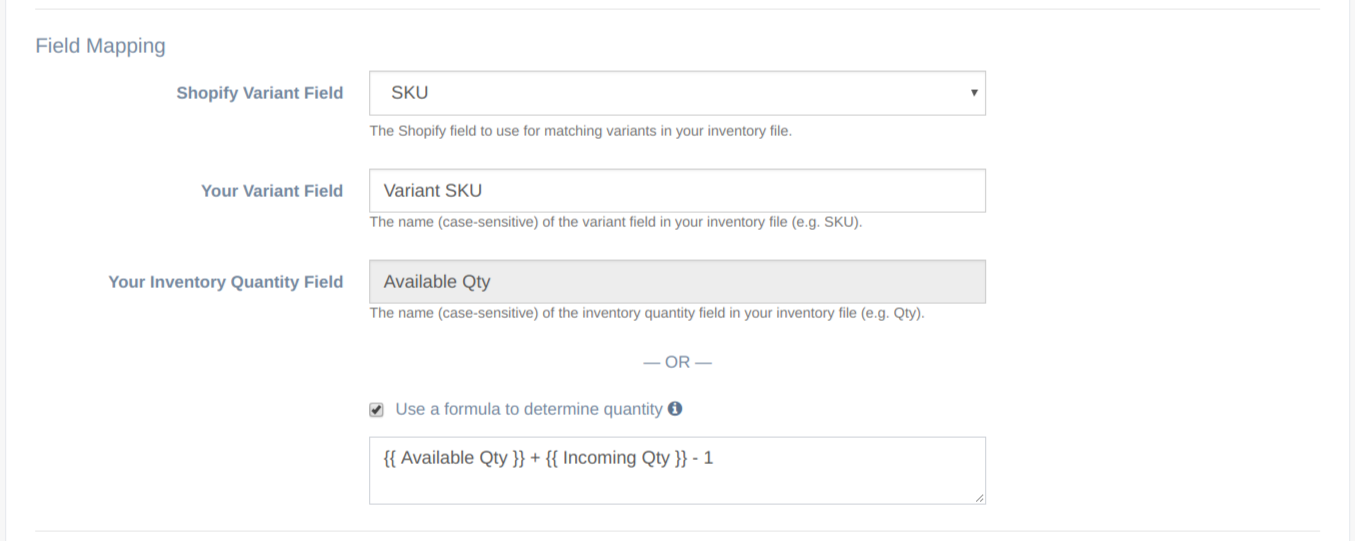## Using a Formula to Determine Inventory Quantity

EZ Inventory supports formulas, giving you much more flexibility for determining the inventory quantity to assign to your products/variants in Shopify.

### What can you do with quantity formulas?

• Set a fixed value to your inventory quantity.
• Perform arithmetic operations based on values from one or more fields in the inventory feed.
• Use conditional logic to determine the quantity if your supplier's feed doesn't provide an exact number for the stock level.
• Strip out unnecessary characters from the values.### Examples

Below are some sample formulas for different use cases where this feature would be useful.

#### 1. Set a fixed value.

There may be cases where you simply want to assign a fixed quantity to your inventory, such as if you want to bulk update a set of products and make them go out of stock. In this case, simply enter a number in the formula field.

```0
```

#### 2. Calculation based on one or more fields in the data.

This is actually a real use case from one of our customers. The system that his warehouse uses to generate the data feed doesn't have the flexibility to add a new field for the quantity that should be used for his Shopify store, even if it can be computed by simply subtracting the values from 2 columns already included in the CSV file.

Our app solves this problem by allowing our users to do arithmetic operations using values from one or more columns in the CSV file.

```{{ QTY ON HAND }} - {{ SOLD }}
```
```{{ available }} + 1
```

#### 3. Using conditional logic when the feed doesn't use actual numbers for the stock level.

This is an interesting use case we've found while doing research on issues Shopify store owners encounter when managing their inventory.

Some suppliers or dropshippers, for example, don't use numbers in their data feeds for the stock level. They may simply use something like a traffic light system with the values "GREEN", "ORANGE", "RED", basically telling the seller that the item availability is high, medium, or out of stock.

Shopify doesn't allow setting stock levels this way, you'll need to assign an actual number for your Shopify store. Our app solves this problem by letting you use conditional logic so you can decide which number to assign to which stock level status.

For example, if the stock level for an item is "GREEN", you might decide to set the quantity for that item to 1000. If it's "ORANGE", then assign 500. Finally, if it's "RED" make the item out-of-stock by setting the quantity to 0.

Here's the formula for that:

```1000 if {{ STOCK LEVEL }} == "GREEN" else 500 if {{ STOCK LEVEL }} == "ORANGE" else 0 if {{ STOCK LEVEL }} == "RED" else "no match"
```

#### 4. Set the quantity in Shopify to 0 if the quantity in the supplier's inventory feed is less than 5.

There may be cases where you want to set the quantity in Shopify to 0 ahead of time when your supplier's stock level is low to reduce the chances of selling an out-of-stock item.

For example, your supplier might only update their feed once a day and an item might've already gone out of stock at some point during the day after that feed was generated, but you won't get the latest data until the next day.

```0 if {{ Quantity }} < 5 else {{ Quantity }}
```

The above formula will set the quantity in Shopify to 0 if the "Quantity" field/column in the inventory feed is less than 5.  Otherwise, it will use the original quantity as shown in the feed.

#### 5. Removing unnecessary characters from the data.

Your inventory feed might include additional characters in the stock level. For example, it might have a column called "Stock" where instead of just having the stock level as numbers, it might say something like:

"250 + more coming soon!"

Since that's not a value that Shopify will accept for the quantity, you can use a formula like this to automatically remove them:

```str({{ Stock }}).replace(" + more coming soon!", "")
```

The formula above just replaces the " + more coming soon!" part with an empty value, which essentially removes it from the data.

#### 6. Coerce the data to an integer and round it down.

Shopify will only accept integer values for the quantity, decimal values will be rejected.  You can use the `int()` function to coerce a decimal value to an integer and round it down at the same time.

For example, you might have a column called "Stock" in your feed and you want to divide this by 4.  So if the Stock is 15, dividing it by 4 will output 3.75.  Using the `int()` function will round this down to 3:

`int({{ Stock }} / 4)`

#### 7. Advanced parsing of multiple values in one column.

This is an actual use case that one of our customers ran into.  Their stock feed provides multiple quantity values in one column separated by a comma and preceded by the location code like this:

"WH1=8,WH2=15,WH3=100"

So we need a formula here to pull down the quantity in WH1, WH2, or WH3.  In their case, they needed to create 3 separate Data Feeds, one for each location, and then use a formula to pull the corresponding quantity by location.

Here's the formula we came up with (where "StockByLocation" is the column name):

```{{ StockByLocation }}.split("WH1=", 1).split(",") if "WH1=" in {{ StockByLocation }} else 0
```

There are probably more use cases out there. If you need assistance coming up with a formula for your store, please don't hesitate to reach out!

Related Articles:

App: EZ Inventory